# 2893. 数据密度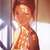unsigned char a = str[i]; //将字符所占字节的内容移入等大的空间中，而这个类型将同样的数据理解为自然数
(int)a;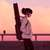#include <iostream>
#include <bitset>
#include <string>

using namespace std;

int gcd(int a, int b) { return !b ? a : gcd(b, a % b); }

int main()
{
int n;
string str;

cin >> n;
while (cin.get() != '\n');

while (n--) {

getline(cin, str);
/*cout << "str = " << str << endl;*/

int sum = 0;

for (auto& item : str) {
auto ptr = new bitset<8>(item);
sum += ptr->count();
/*cout << "sum = " << sum << endl;*/

}

int g = gcd(sum, str.length() * 8);
cout << sum / g << '/' << str.length() * 8 / g << endl;

}

return 0;

}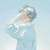### include

using namespace std;

int p,q;
char strs;

int gcd(int aa,int bb)
{
if(aa<bb) swap(aa,bb);
if(bb==0) return aa;
else return gcd(bb,aa%bb);
}
void solve(char aa)
{
p+=8;
for(int i=1;i<=8;i++)
if(aa&((1<<(i-1)))) q++;
return;
}
int main()
{
int n;
scanf(“%d”,&n);
gets(strs);
for(int step=0;step<n;step++)
{
gets(strs);
int ll=strlen(strs);
p=q=0;
for(int i=0;i<ll;i++) solve(strs[i]);
while(gcd(p,q)!=1)
{
int tmp=gcd(p,q);
p/=tmp;
q/=tmp;
}
printf(“%d/%d\n”,q,p);
}
return 0;
}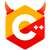#include <cstdint>
#include <iostream>
#include <numeric>
#include <string>
using namespace std;

inline unsigned count_ones(uint8_t _num) {
unsigned ret = 0;
while (_num) {
ret += _num & 1;
_num >>= 1;
}
return ret;
}

int main() {
int n; cin >> n; cin.get();
for (int i = 0; i < n; ++i) {
string a; getline(cin, a);
auto bits = a.size() * sizeof(char) * 8;
decltype(bits) ones = 0;
for (auto ch : a)
ones += count_ones(*reinterpret_cast<uint8_t *>(&ch));
auto div = gcd(bits, ones);
cout << ones / div << '/' << bits / div << '\n';
}
}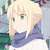### include

using namespace std;
int gcd(int x , int y){
if(!y) return x;
else return gcd(y , x%y);

}
int main()
{
int N;
cin >> N;
cin.get();
while(N–){
string str;
getline(cin, str);
int a = 0, len = str.size();
for(int i = 0;i < len;i++){
for(int j = 0;j < 8;j++){
if(str[i] % 2) a++;
str[i] >>= 1;
}
}
int temp = gcd(a, 8len);
cout << a/temp << “/” << 8
len/temp << endl;
}
}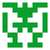### include

void main()
{
int n,i,j,k,l,m;
char s;

scanf("%d",&n);
getchar(); //用getchar把空格读掉。
for(i=0;i<n;++i)
{
l=0;
gets(s);
j=strlen(s);
for(k=0;k<j+1;++k)
{
l=l+judge(s,k);
}
m=gcd(l,j*8);
printf("%d/%d\n",l/m,(j*8)/m);

}


}
judge(char s[],int k)
{
int i,m,n;
m=0;
if(s[k]<0)i=s[k]+256;
else i=s[k];
if(i>=128){m++;i=i-128;}
if(i>=64){m++;i=i-64;}
if(i>=32){m++;i=i-32;}
if(i>=16){m++;i=i-16;}
if(i>=8){m++;i=i-8;}
if(i>=4){m++;i=i-4;}
if(i>=2){m++;i=i-2;}
if(i>=1){m++;i=i-1;}

return m;


}
int gcd(int a,int b)//求两个数的最大公约数
{
if(a==0)
{
return b;
}else
{
return gcd(b % a,a);
}
}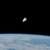#include<iostream>
#include<cstdio>
using namespace std;
int count(const string &s);
int gcd(int a, int b);

int main() {
int n;
cin>>n; getchar();
string s;
for(int i=0; i!=n; ++i) {
getline(cin, s);
int ones=count_ones(s), len=8*s.size(), g=gcd(ones,len);
cout<<ones/g<<"/"<<len/g<<endl;
}
return 0;
}

int count_ones(const string &s) {
int cnt=0;
for(auto i:s) {
int n=i;
if(n<0) n=256+n;    // 不知道为什么有负数
while(n!=0) { cnt += (n&1); n>>=1; }
}
return cnt;
}
int gcd(int a, int b) {
if(a%b==0) return b;
return gcd(b, a%b);
}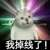#include<bits/stdc++.h>
using namespace std;

int char2count(char a) {
int i = a;
bitset<8> temp(i);
return temp.count();
}

void solve(string str) {
int fenzi = 0;
for(int i = 0; i < str.length(); ++i) {
fenzi += char2count(str[i]);
}
int fenmu = str.length()*8;
int gcd = __gcd(fenzi, fenmu);

printf("%d/%d\n",fenzi/gcd,fenmu/gcd);
}

int main() {
int num;
cin >> num;
cin.get();
for(int cas = 0; cas < num; ++cas){
string str;
getline(cin, str);
solve(str);
}
}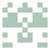int gy(int a, int b) {
if (b == 0)
return a;
return gy(b, a % b);
}

int main() {
int n;
cin >> n;
getchar();
while (n–) {
string s;
int count1 = 0, count2 = 0;
getline(cin, s);
for (int i = 0; i < s.size(); i++) {
for (int j = 0; j < 8; j++) {
if (s[i] % 2 == 1)
count1++;
s[i] >>= 1;
}
}
count2 = 8 * s.size();
int temp = gy(count2, count1);
cout << count1/temp << “/” << count2/temp << endl;
}
return 0;
}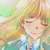#include <iostream>
#include <string>
#include <cstdio>
using namespace std;
int gcd(int a, int b)
{
return(b==0)?a:gcd(b,a%b);
}
int main()
{
int n, i, j;
string s;
cin >> n;
getchar();
while(n--)
{
getline(cin, s);
int len = s.size(), cnt = 0, G;
for(i = 0; i < len; ++i)
{
int x = 1;
for(j = 0; j < 8; ++j)
{
if(s[i] & x) cnt++;
x = x << 1;
//cout << x << endl;
}
}
G = gcd(cnt, len * 8);
printf("%d/%d\n", cnt/G, 8*len/G);
}
return 0;
}